# Covid19 Correlations Forecast

Web app with interactive forecasts based on correlations
Alternatives To Covid19 Correlations Forecast
Select To Compare

# Interactive Covid-19 Forecast and Correlation Explorer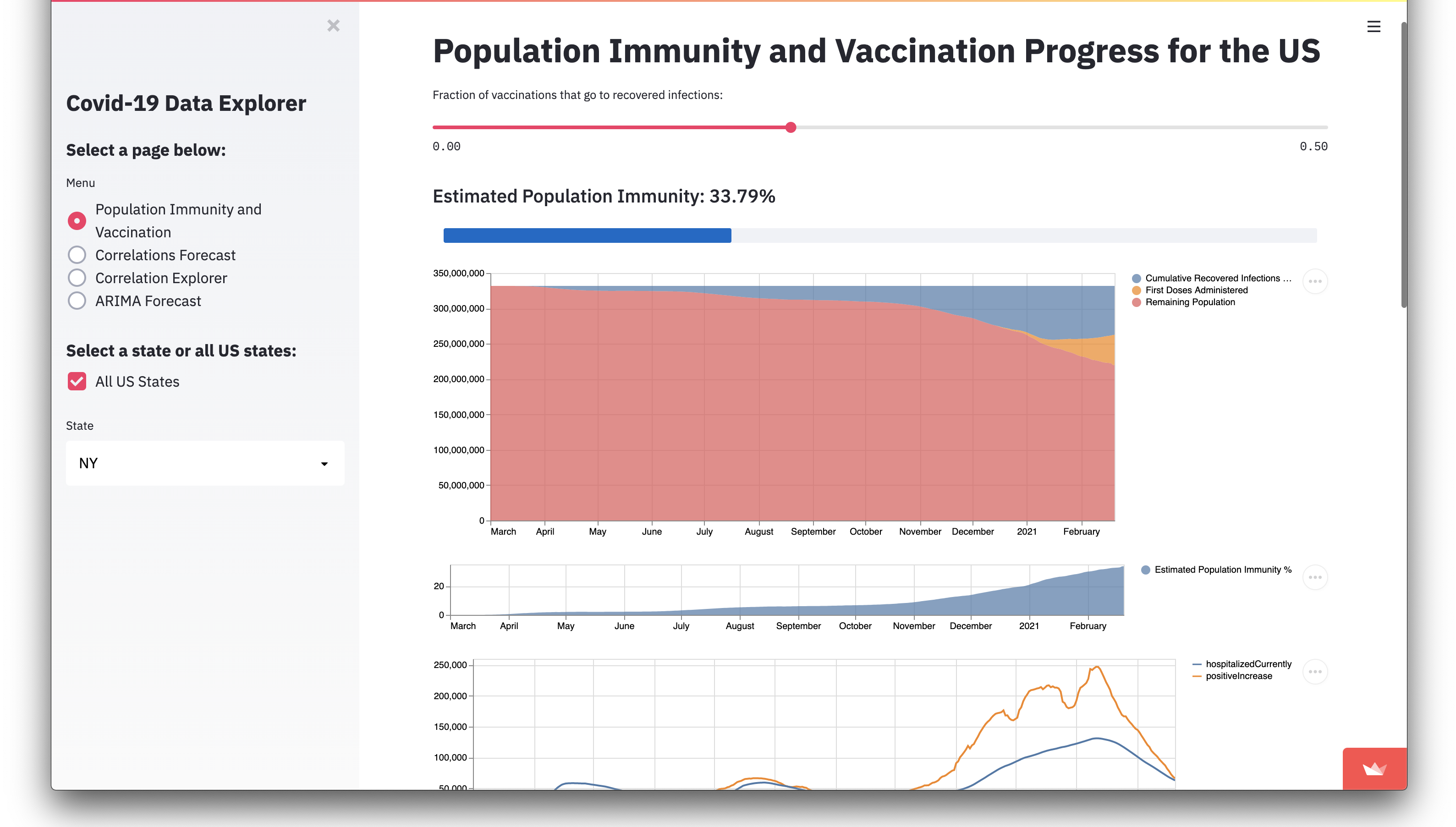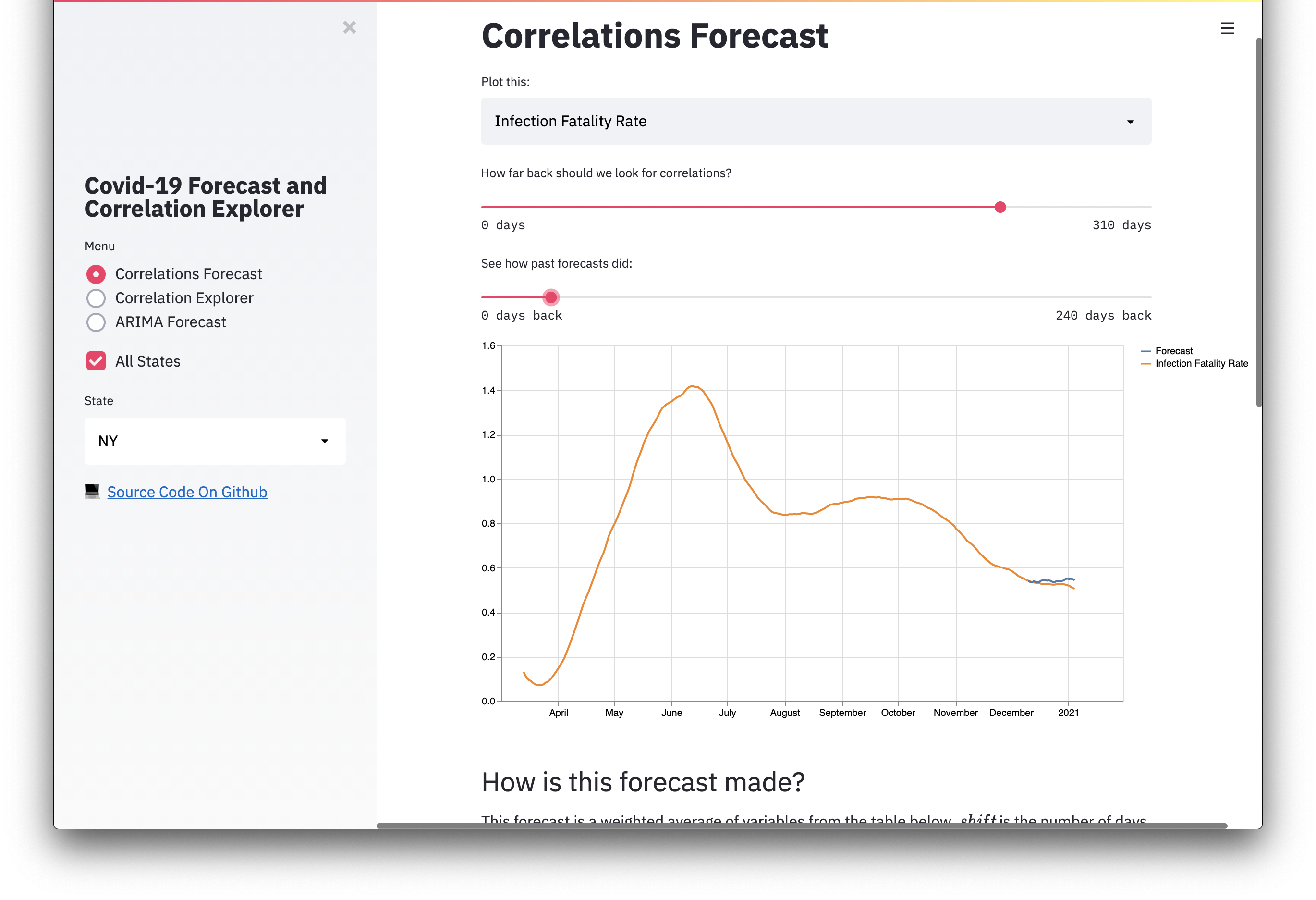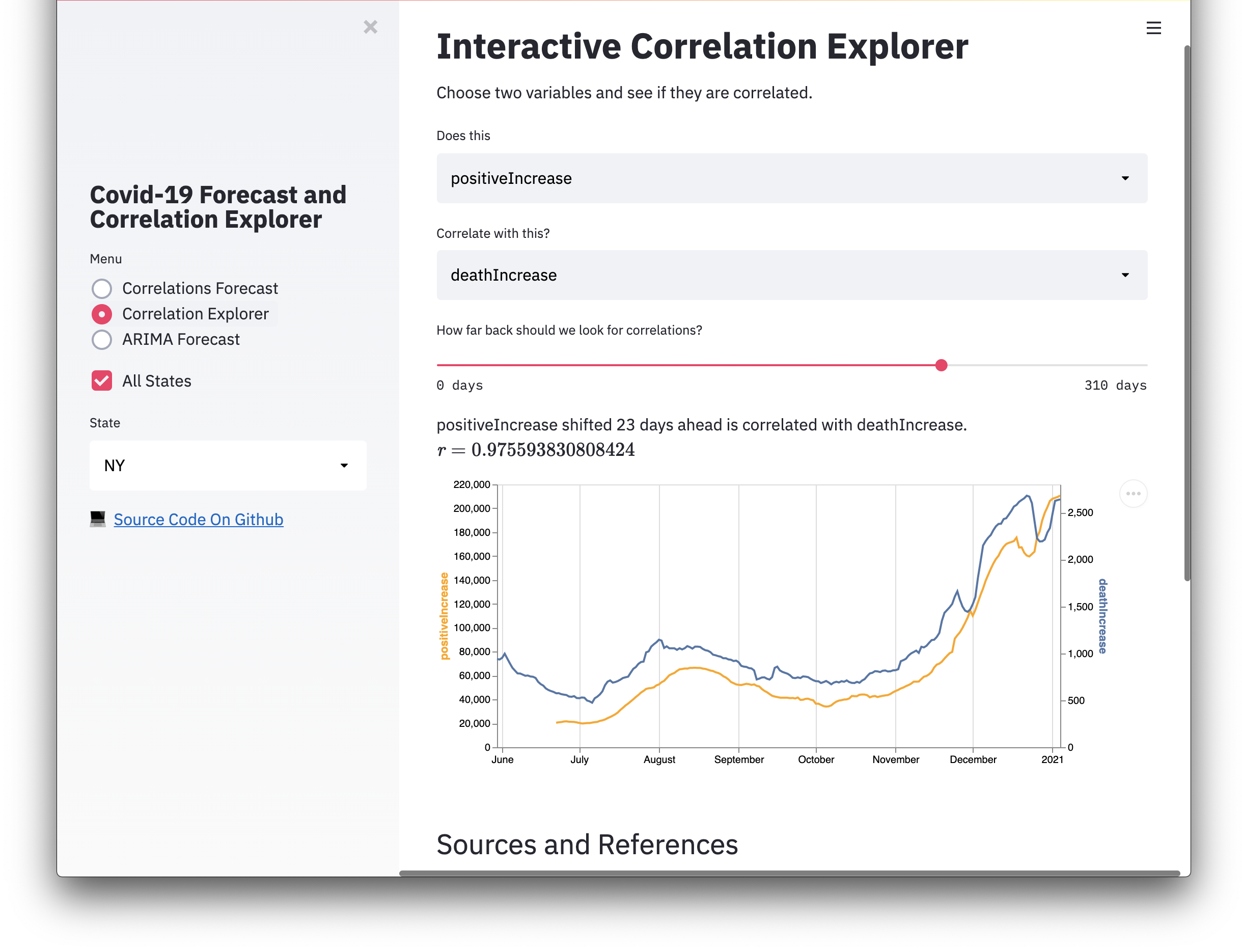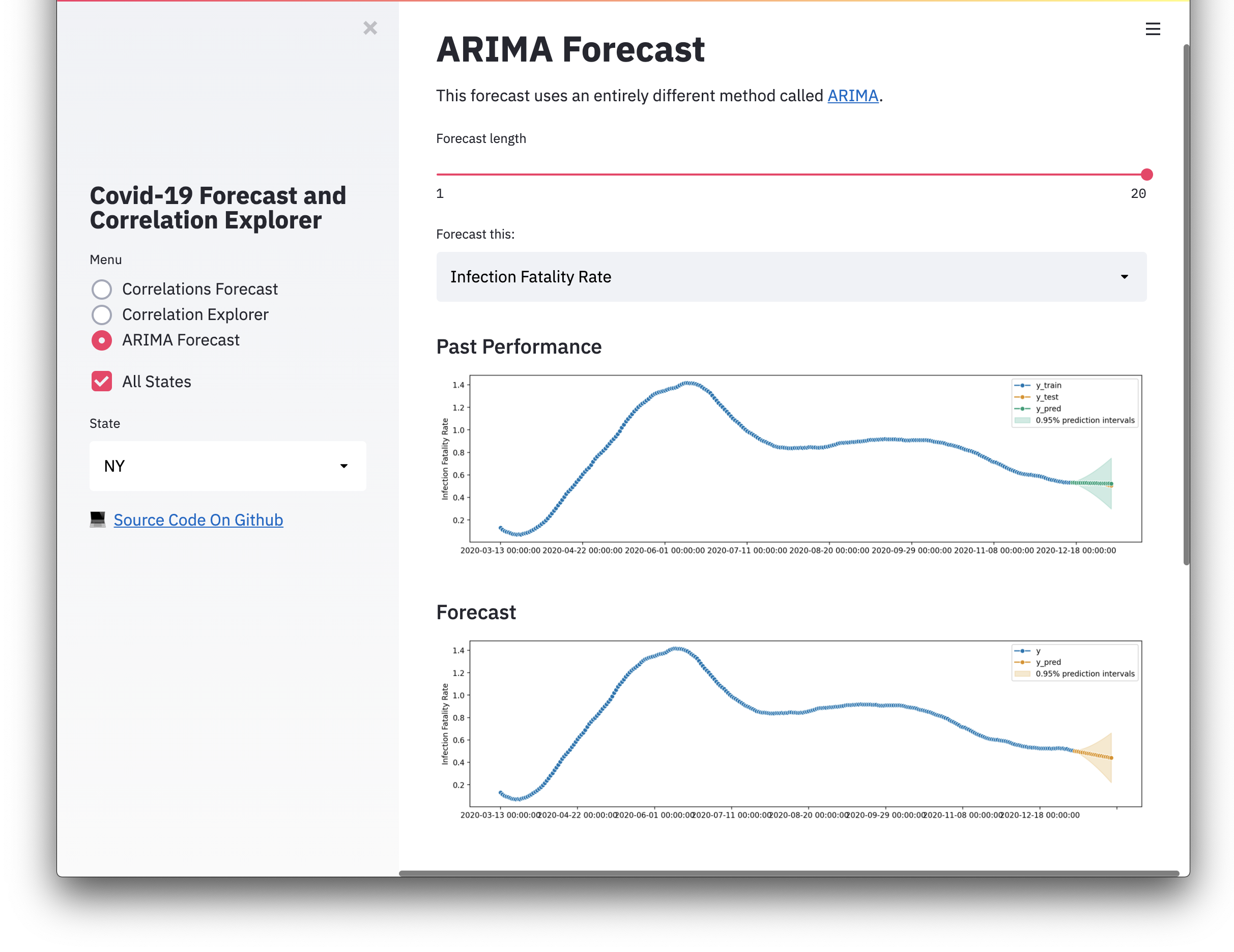## How is this forecast made?

This forecast is a weighted average of correlated variables. shift is the number of days a is shifted forward, and r is the Pearson correlation coefficient between shifted a and b.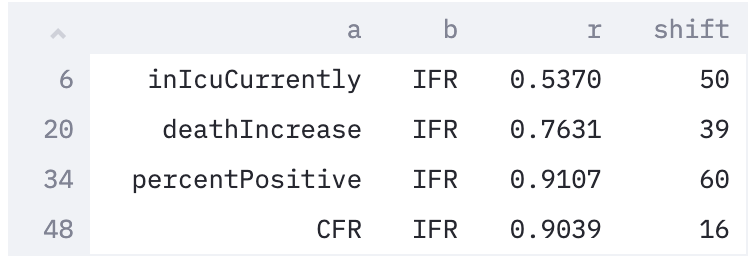The model searches every combination of a, b, and shift for the highest r values. Only correlations >0.5 are used. r is used to weight each component of the forecast, and each component is scaled and aligned to the forecasted variable b. The forecast length is the average shift weighted by the average r.

Ordinary Least Squares regression is also used to scale each series from the a column as well as the final forecast.

You can choose two variables and see if they are correlated.

Infection fatality rate is calculated using the formula described by https://covid19-projections.com/estimating-true-infections-revisited.

Data is pulled daily from https://covidtracking.com

# To Do

• Score forecasts with MSE or other metric

• Feed correlated variables into ML regression model for forecasting

• PCA, cluster, and TSNE plot different states - In progress

• ARIMA forecast

• Try using cointegration instead of correlation

• Cleanup code

• Intra-state correlations

Popular Applications Categories
Related Searches

Get A Weekly Email With Trending Projects For These Categories
No Spam. Unsubscribe easily at any time.
Python
Forecast